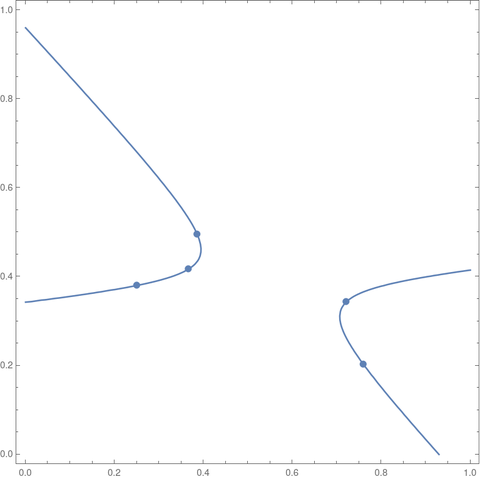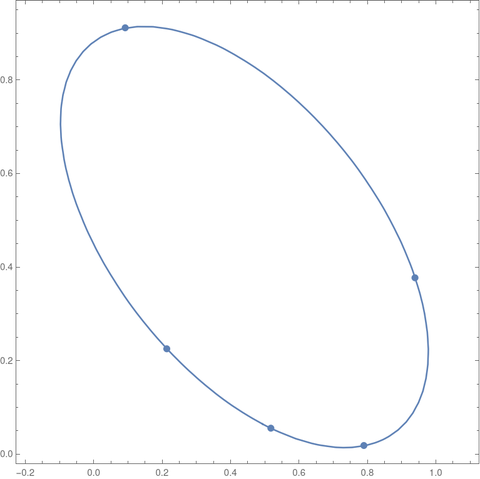# Equation of an ellipse or hyperbola through five points

Yesterday I wrote about the equation of a circle through three points. This post will be similar, looking at the equation of an ellipse or hyperbola through five points. As with the earlier post, we can write down an elegant equation right away. Making the equation practical will take more work.

The equation of a general conic section through five points## Direct but inefficient solution

The most direct (though not the most efficient) way to turn this into an equationis to expand the determinant by minors of the first row.## More efficient solution

It would be much more efficient to solve a system of equations for the coefficients. Since the determinant at the top of the page is zero, the columns are linearly dependent, and some linear combination of those columns equals the zero vector. In fact, the coefficients of such a linear combination are the coefficients A through F that we’re looking for.

We have an unneeded degree of freedom since any multiple of the coefficients is valid. If we divide every coefficient by A, then the new leading coefficient is 1. This gives us five equations in five unknowns.## Python example

The following code will generate five points and set up the system of equations Ms = b whose solution s will give our coefficients.

    import numpy as np

np.random.seed(20230619)

M = np.zeros((5,5))
b = np.zeros(5)
for i in range(5):
x = np.random.random()
y = np.random.random()
M[i, 0] = y**2
M[i, 1] = x*y
M[i, 2] = x
M[i, 3] = y
M[i, 4] = 1
b[i] = -x*x


Now we solve for the coefficients.

    s = np.linalg.solve(M, b)
A, B, C, D, E, F = 1, s, s, s, s, s


Next we verify that the points we generated indeed lie on the conic section whose equation we just found.

    for i in range(5):
x = M[i, 2]
y = M[i, 3]
assert( abs(A*x*x + B*y*y + C*x*y + D*x + E*y + F) < 1e-10 )


## Ellipse or hyperbola?

It turns out that the five points generated above lie on a hyperbola.If we had set the random number generator seed to 20230620 we would have gotten an ellipse.When we generate points at random, as we did above, we will almost certainly get an ellipse or a hyperbola. Since we’re generating pseudorandom numbers, there is some extremely small chance the generated points would lie on a line or on a parabola. In theory, this almost never happens, in the technical sense of “almost never.”

If 4ABC² is positive we have a an ellipse. If it is negative we have a hyperbola. If it is zero, we have a parabola.

## 2 thoughts on “Equation of an ellipse or hyperbola through five points”

1. Richard Pennington

Note that in your hyperbola example one point (the middle point on the LH branch) lies inside the convex quadrilateral defined by the other four points. So no ellipse can pass through these five points. In the second example the pentagon defined by the five points is convex.

2. Dave Neary

Do two crossing lines count as a degenerative hyperbola, I wonder?